# Scientific Writing

## Grammar and Style

• Training set, validation set, test set.

• Use of “SOTA”. “State of the art” as a noun is written without hyphens, as in “Our algorithm represents the state of the art”. As an adjective, “start-of-the-art” is hyphenated, as in, “These are state-of-the-art results”. Also, don’t forget the determiner in the nominal form, i.e., the state of the art.

• Related work. “Related work”, not “related works”. “Work” is a collective noun, like “staff”. You don’t write “research staffs”.

• That vs Which. The meaning of the sentence is not properly conveyed if the that clause is not included. If you remove the which clause from the sentences, the meaning of each sentence is still conveyed. See detail in here

• Resolving ambiguity using ‘and’:

• To my parents, Ayn Rand and God. This is ambiguity because “Ayn Rand and God” can be read as in apposition to my parents. A comma before and removes the ambiguity. To my parents, Ayn Rand, and God. See detail in Serial comma

# LaTeX Tips

## Appearance

### Author

\author{
\begin{tabular}{c@{\ \ \ }cc}
Xu Liang & Yasufumi Taniguchi & Hiroki Nakayama \\[3pt]
TIS Inc. & TIS Inc. & TIS Inc. \\[3pt]
% \multicolumn{3}{c}{TIS Inc. TIS Inc.} \\
\vspace{-4ex}
\end{tabular}}
\date{\texttt{\{ryo.sho, taniguchi.yasufumi, nakayama.hiroki\}@tis.co.jp}}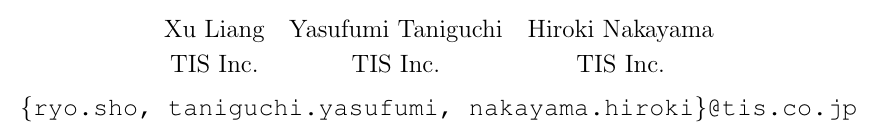### Math Function

• Conditional Probability : $P(x \mid y)$, ues P(x \mid y) , instead of P(x | y)
• Adding equation number only if we want to cite it in other place.
# turn on equation numbering
\begin{equation} \label{eu_eqn}
coverage = \frac{\sum \delta (e_i, L)}{\sum_{i=0}^{n}e_{i}},
\end{equation}

# turn off equation numbering
\begin{equation*} \label{eu_eqn}
coverage = \frac{\sum \delta (e_i, L)}{\sum_{i=0}^{n}e_{i}},
\end{equation*}

### Code

Using verbatim or listing:

\begin{verbatim}
your
code
example
\end{verbatim}

### Footnote & URL

• Footnotes go after the punctuation, as in End of sentence.\footnote{...}. Note, there is no space between punctuation and the footnote. And it also go after the closing parenthesis. See detail here.
• Use \url for URL representation.
\usepackage{hyperref}

\noindent \textbf{Data}~In our experiments, we use two Japanese annotated corpora, the Balanced Corpus of Contemporary Written Japanese~(BCCWJ)\footnote{\url{https://pj.ninjal.ac.jp/corpus_center/bccwj/}}, and Mainichi Newspaper Corpus.\footnote{\url{http://www.nichigai.co.jp/sales/mainichi/mainichi-data.html}} According to the entity annotation scheme,\footnote{\url{https://nlp.cs.nyu.edu/ene/ene_j_20160801/Japanese_7_1_2_160917.htm}} these datasets contains multiple entity types. But we only extract the samples that contain the Company" type. There are total 4,391 sentences from two datasets~(see Table~\ref{t:dataset}).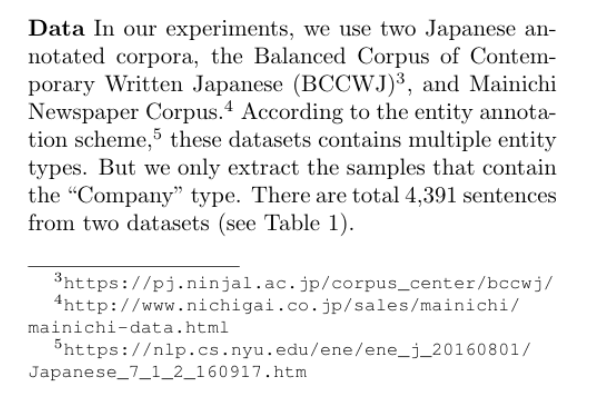### Quotation

Double quotation in latex:

The Company'' type is ...

### Table

Use LaTeX Tables Generator to generate the table, and insert it in the between \begin{tabular} and \end{tabular} .

One column table begin with table , and resizebox(0.45\textwideth) could resize the table size, and use the \centering to center the words:

\begin{table}[t]
\centering
\resizebox{0.45\textwidth}{!}{
\begin{tabular}
# insert the tabular here
\end{tabular}
}
\caption{Lexicons statistics}
\label{t:lexicon}
\end{table}

Two column table begin with table* . Do not use \vspace{-0.65cm} to adjust the space, avoiding dest reject. If the space is not enough, you could read your paper few times and delete some reduent content.

\begin{table*}[]
\centering
\begin{tabular}{|c|c|c|c|c|c|}
# insert the tabular here
\end{tabular}
\caption{Intrinsic evaluation ...}
\label{t:coverage}
% \vspace{-0.65cm}
\end{table*}

A desk reject means that the program chairs (or editors) reject a paper without consulting the reviewers. This is done for papers that fail to meet the submission requirements, and which hence cannot be accepted. Filtering out desk rejects in advance is common practice for both conferences and journals.

Fix table position, let the table under text.

\usepackage{placeins}

\FloatBarrier
\begin{table}[h]
\resizebox{0.45\textwidth}{!}{
\centering
\begin{tabular}
# insert the tabular here
\end{tabular}
}
% \caption{Alias generation process}
% \label{t:alias}
% \vspace{-0.65cm}
\end{table}
\FloatBarrier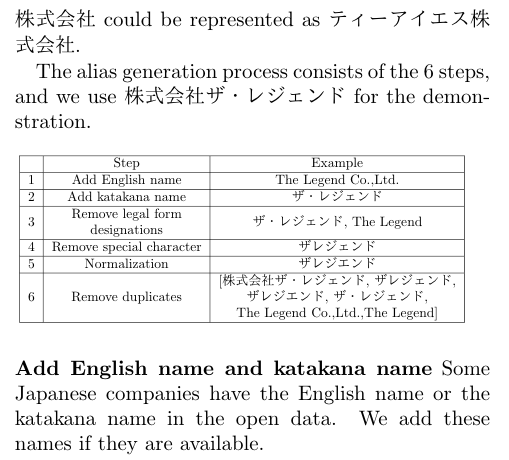Table alignment: the text in the left 1-th column should be left aligned, and the numbers in the right column should be right aligned: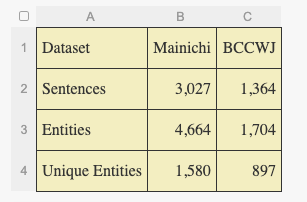### Refer to a figure or table

There is no need to add ‘the’ before Table~\ref{t:coverage}.

Table~\ref{t:coverage} list the coverage scores for different lexicons and datasets. We can see the JCL lexicon cover most company names~(0.5082 in Mainichi and 0.5407 in BCCWJ) than other single lexicon. As for the multiple lexicons, JCL also boost the coverage score of IPAdic-NEologd~(from 0.5291 to 0.6923 in Mainichi, from 0.4593 to 0.6522 in BCCWJ).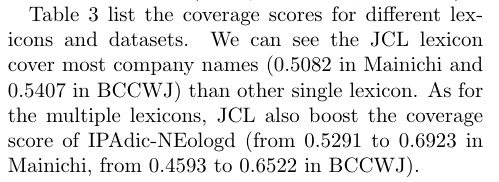### Citation

• Cite a reference as Lin et al.~\cite{Lin}. Note ~ prevents ugly breaks.

Blog: BrambleXu
GitHub: BrambleXu
Medium: BrambleXu

目录